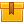# Introduction to Statistical Process Control (A8501)

## Prerequisites

This lesson is designed so that no prior knowledge is required. However, knowledge of basic mathematical skills is recommended.

## Description

This lesson introduces statistical process control (SPC) as a prevention method used to reduce quality costs. The lesson explains variability and shows how to construct a histogram to represent it. The normal curve is described as well as its probability and relevance to the philosophy of never-ending improvement.

## Objectives

Explain the difference between prevention and detection systems. Define statistical process control and quality as it relates to SPC. Describe the various classifications of quality costs and discuss their relevance to SPC. Describe the concept of variation. Generate frequency charts and histograms. Apply basic SPC terminology such as variable, attribute, class width, and frequency. Describe what is meant by probability ? Show how normal curves can represent measured quantities.

## ContentThis lesson introduces statistical process control (SPC) as a prevention method used to reduce quality costs. The lesson explains variability and shows how to construct a histogram to represent it. The normal curve is described as well as its probability and relevance to the philosophy of never-ending improvement.

•Introduction to Statistical Process Control

0
0 Reviews